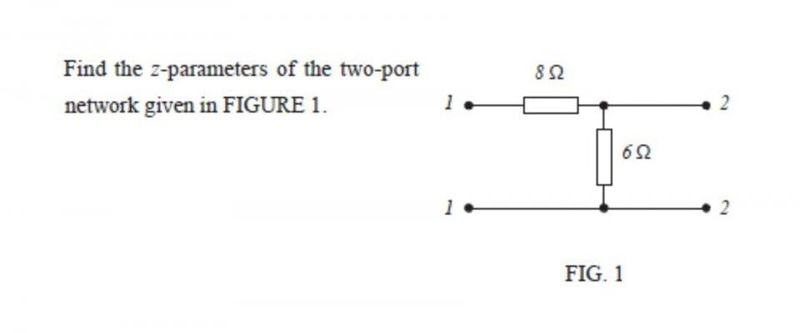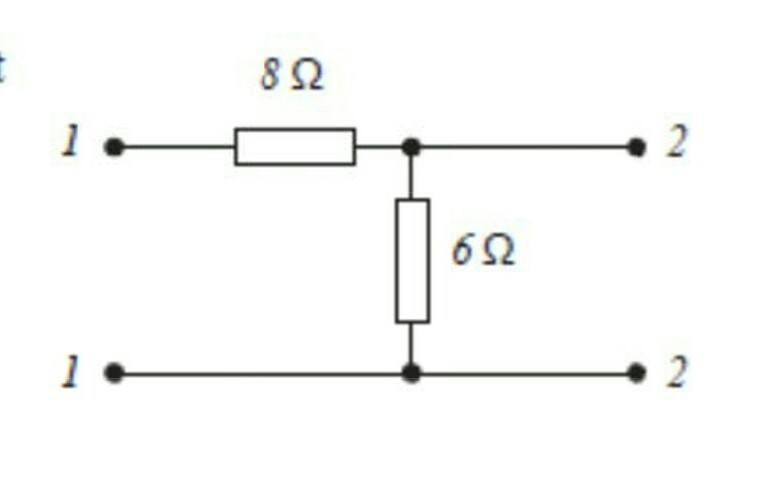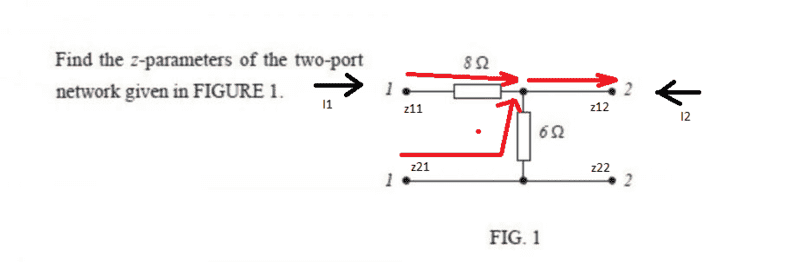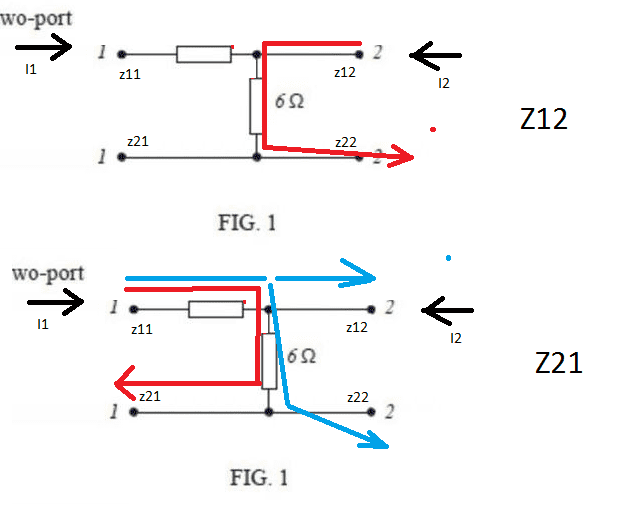# Two-port network z-parameters

• acw260

## Homework StatementAs above.

## The Attempt at a Solution

(z11) With the output open circuit (and therefore I2=0) z11 = V1 / I1 = (R8 + R6)I1 / I1 = 14ohms;

(z12) With the input open circuit (and therefore I1=0) z12 = V1 / I2 = R6 = 6ohms;

(z21) With the output open circuit (and therefore I2=0) z21 = V2 / I1 = V1 x (R6 / (R8+R6) ) x ( (R8+R6) / V1) = R6 = 6ohms;

(z22) With the input open circuit (and therefore I1=0) z22 = V2 / I2 = R6xI2 / I2 = R6 = 6ohms

Is the above correct? It seems so easy that I'm worried I've overlooked something and made a really basic mistake!

#### Attachments

• Document1.pdf
48.3 KB · Views: 1,000
Last edited by a moderator:

Hello acx, welcome to PF :)

Some things are easy :) enjoy while it lasts...

•scottdave
Thanks BvU, I shall do just that!

Where does the value for ##I1## come from? In the first equation for ##z11## where ##I2=0## there appears to be a value of 1 for ##I1##. Then in the next equation for ##z12## and ##I1 =0## there appears to be a value of 1 for ##I2##. How are these values derived please as I can't seem to work out and my notes tell me virtually nothing. Appreciated thanks

Where does the value for ##I1## come from? In the first equation for ##z11## where ##I2=0## there appears to be a value of 1 for ##I1##. Then in the next equation for ##z12## and ##I1 =0## there appears to be a value of 1 for ##I2##. How are these values derived please as I can't seem to work out and my notes tell me virtually nothing. Appreciated thanks
Can you enlarge your screen or change the default font to see print more clearly?

z11 = V1 / I1 = (R8 + R6) I1 / I1 = 14ohms

The formulae come from applying the z-parameter equations characterising a 2 port network.

•scottdave
For z11 assume that the input is not open circuit. Therefore I1 is not equal to zero. I1 then cancels out from the equation leaving z11 = R8+R6. You don't need a value for I1, just that it doesn't equal zero.

For z12 again it doesn't matter what value you have for I2. You show that z12 = R6 = 6ohms. The value of I2 becomes irrelevant.

OK thanks for the info.

So as I understand this ##Z11## is the impedance seen looking into port 1 when port 2 (output) is open
.

Output is open circuit so ##I2=0##

##Z11=\frac{V1}{I1}=\frac{(R8+R6)(I1)}{I1}## The ##I1## cancels leaving ##R8+R6=14\Omega##

So for ##Z22## is the impedance looking into port 2 when port 1 (input) is open

Input is open circuit so ##I1=0##

##Z22=\frac{V2}{I2}=\frac{(R6)(I2)}{I2}## The ##I2## cancels leaving ##R6=6\Omega##

The reason ##Z22## only has ##R6## to deal with is because looking into the network from port 2 we can only see the ##6\Omega## resistance

So for ##Z12## i understand this is the ratio of the voltage at port 1 to the current at port 2 when port 1 is open. If port 1 is open the ##I1=0##

You have used the equation ##Z12 =\frac{V1}{I2} = R6 = 6\Omega##

My question is why does ##V1=6\Omega## this time whereas when for ##Z11,V1=R8 + R6##

Thanks

You have used the equation ##Z12 =\frac{V1}{I2} = R6 = 6\Omega##

My question is why does ##V1=6\Omega## this time whereas when for ##Z11,V1=R8 + R6##
Inject current at top terminal 2 and it exits at lower terminal 2. With port 1 open, determine V1.So current will flow through ##R6## and back out via the lower terminal 2 because port 1 is open. So R8 does not come into the equation so to speak??

I have a better understanding of this now, yes. Thanks for the help

•NascentOxygen
12!
Sneak extra info: for a passive network, x12 = x21. x can be either z or y parameters. (The rules for h, a, s parameters differ slightly).

## Homework Statement

View attachment 112127

As above.

## The Attempt at a Solution

(z11) With the output open circuit (and therefore I2=0) z11 = V1 / I1 = (R8 + R6)I1 / I1 = 14ohms;

(z12) With the input open circuit (and therefore I1=0) z12 = V1 / I2 = R6 = 6ohms;

(z21) With the output open circuit (and therefore I2=0) z21 = V2 / I1 = V1 x (R6 / (R8+R6) ) x ( (R8+R6) / V1) = R6 = 6ohms;

(z22) With the input open circuit (and therefore I1=0) z22 = V2 / I2 = R6xI2 / I2 = R6 = 6ohms

Is the above correct? It seems so easy that I'm worried I've overlooked something and made a really basic mistake!

Hi, sorry to be this a pain as I know this thread is a couple of years old but I'm now working through the same question, was just wondering if anyone could explain why for z21 V2 was changed to V1 x (R6 / (R8+R6) ) x ( (R8+R6) / V1) and how that equals 6 ohms? I'm looking to understand this as I don't want to 'just know the answer'. Is this because the R8 & R6 are effectively in parallel like the attached drawing?

Also is this approach also acceptable?
Using the formulas V1 = z11*I1+z12*I2 and also V2 = z21*I1+Z22*I2
For inpute open:
Z22 = V2 / I2 = (Z*I2) / I2 = z22 = 6 ohms
For output open:
z11= V1 / I1 = (Z*I1) / I1 = 8+6 = Z11 = 14 ohms

I am happy with the above as the the formula rearranges nice and easily however with the following two I end up with the 'wrong V over the wrong I'. Unsure of how to work around this without values for V or I
Z12 = V1 / I2 =
Z21= V2 / I1 =

Appreciate any help!#### Attachments

Last edited:
z12 = v1/i2 |i1=0
z21 = v2/i1 |i2=0

what do you compute?

(Fact: in any passive 2-port network, z12 = z21). If you have any active element in your network like a transistor, this does not necessarily hold).

Okay so if z12 = z21
V1 / I2 = V2 / I1

which I could rearrange to
V1 * I1 = V2 * I2
V1 = (V2*i2) / I1 & V2 = (V1*I1) / I2
and
I1 = (V2*I2) / V1 & I2 = (V1*I1) / V2
so
I1 = (V2*I2) / ((V2*I2) / I1)
I1*I1 = (V2*I2) / ((V2*I2)
I1*I1 = (Z*I2*I2) / ((Z*I2*I2)
I1*I1 = 0 ?

V1 = (V2*i2) / I1 & V2 = (V1*I1) / I2
V1 = (((V1*I1) / I2)*i2) / I1 = (V1*I1) / I1 = V1 ?

z12 = ((V2*i2) / I1) / ((V1*I1) / V2)
z12 = (V2*i2) / I1) * (V2 / (V1*I1))
z12 = (I2*V2^2) / (V1*I1^2)

Safe to say I'm confused haha

To try and understand the current flow path, I think I understand why Z12 = 6ohms as it passes only through the 6ohm resistor ( first crude drawing )
I would've though that Z21 = 14ohms as well as it would take the red path ( second drawing), can't quite understand why it is 6ohms unless it takes the blue path, meaning that the 8 & 6 are in parallel?#### Attachments

To try and understand the current flow path, I think I understand why Z12 = 6ohms as it passes only through the 6ohm resistor ( first crude drawing )
I would've though that Z21 = 14ohms as well as it would take the red path ( second drawing), can't quite understand why it is 6ohms unless it takes the blue path, meaning that the 8 & 6 are in parallel?
View attachment 237875
Right, the red path. Let's look at the red path.
So z21 = v2/i1 with i2=0. So I run a current i1 into the input, what's the output voltage with the output open?

Sorry, I assume you mean the red path in the second drawing? If so current would flow from top terminal 1 to bottom terminal one, so with both terminal 2s open the output voltage would equal the voltage across the 6 ohm resistor?

Wait, so would:

Z21 = V2 / I1 = (6 * I1) / I1 = 6ohms as I1 cancels out?

Last edited:
Then Z12 = V1/ I2 , the current would act as in the first drawing so V1 = I2 * Z

So:
Z12 = (Z*I2)/ I2 = (6 * I2) / I2 = 6 ohms

If this is the case then I now don't understand why Z11 is 14 ohms rather than the same as Z21 with 6, unless it is because from the perspective from Z21 the 8 ohm resistor is downstream of the 6ohm?

Last edited:
Then Z12 = V1/ I2 , the current would act as in the first drawing so V1 = I2 * Z
So:
Z12 = (Z*I2)/ I2 = (6 * I2) / I2 = 6 ohms
If this is the case then I now don't understand why Z11 is 14 ohms rather than the same as Z21 with 6, unless it is because from the perspective from Z21 the 8 ohm resistor is downstream of the 6ohm?
Well, you got z12 and z21 right.
So, let's re-examine z11: z11 = v1/i1 with i2 zero (nothing connected to port 2). You put in v1 volts, what do you get for the current?
BTW although I told you about z12 = z21, you shouldn't use that fact until you're comfortable with solving for them with the basic equations. It takes a while and you'd benefit from the double-check. And also BTW you may know there are several other two-port parameters, like yij, hij, aij. You should be able to find those from the respective equations also.

Okay so Z11 = V1 /I1 with V1 volts would mean that current = I1. I think I maybe understand now.

So Z11 = V1 / I1, voltage will run from top terminal 1 to bottom terminal 1 so will. = 14 ohms

However for Z21 = V2 / I1, V2 contacts will be in parallel with the 6 ohm resistor, so Z21 = (Z6+I1)/ I1 = 6 ohms

Okay noted I'll try learn to use the basic ones first.

Okay so Z11 = V1 /I1 with V1 volts would mean that current = I1. I think I maybe understand now.
Z21 = (Z6+I1)/ I1 = 6 ohms
What kind of equation is this?But your answer is right. So are z12 and z21.

Sorry I'm not sure what you mean by type of equation? Basic ohms law or?

Sorry I'm not sure what you mean by type of equation? Basic ohms law or?
Different two-port parameters have different equations.
For example, the y parameters are defined by the equations
i1 = y11 v1 + y12 v2
i2 = y21 v1 + y22 v2

etc. You can look upthe ones for the h or a or other parameters. There is even a set (the "s" parameters) based on incident, transmitted and reflected waves. They're particularly appropriate for microwave and other high-frequency circuits. You won't run into those unless you're going for an EE degree, probably.

I should also mention that all parameters can be complex transforms, i.e. have real and imaginary parts, if there are inductive and/or capacitive components in the network. For example, jωL for an inductor's impedance etc.

What kind of equation is this?But your answer is right. So are z12 and z21.

Yeah I was using V1 = z11*I1+z12*I2 with I2=0 so that V1 = z11*I1 which I rearranged to z11 = V1/I1

Just wondering if you could explain in algebraic terms what the original post of:

z21 = V2 / I1 = V1 x (R6 / (R8+R6) ) x ( (R8+R6) / V1) = R6 = 6ohms;

is?

Last edited: